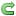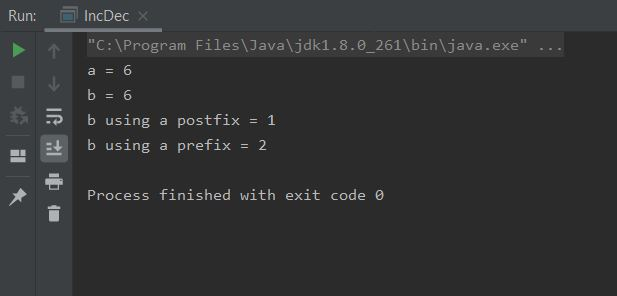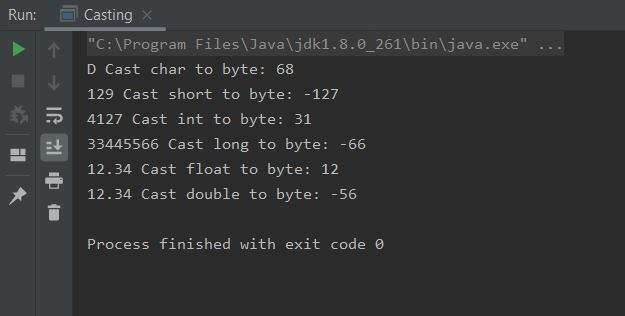Symbols used for mathematical and logical manipulation that are recognized by the compiler are commonly known as operators in Java. In the first of two lessons on operators we look at the arithmetic, relational, logical and assignment operators.

### Arithmetic OperatorsTop

We are familiar with most of the arithmetic operators in the table below from everyday life and they generally work in the same way in Java. The arithmetic operators work with all the Java numeric primitive types and can also be applied to the `char` primitive type.

Operator Meaning Example Result Notes
+Addition`int b = 5; b = b + 5;`10
-Subtraction`int b = 5; b = b - 5;`0
/Division`int b = 7, b = b / 22;`3When used with an integer type, any remainder will be truncated.
*Multiplication`int b = 5; b = b * 5;`25
%Modulus`int b = 5; b = b % 2;`1Holds the remainder value of a division.
++Increment`int b = 5; b++;`6See below.
--Decrement`int b = 5; b--;`4See below.

#### Increment And Decrement Operators

Both these operators can be prepended (prefix) or appended (postfix) to the operand they are used with. When applied to an operand as part of a singular expression it makes no difference whether the increment and decrement operators are applied as a prefix or postfix. With larger expressions however, when an increment or decrement operator precedes its operand, Java will perform the increment or decrement operation prior to obtaining the operand's value for use by the rest of the expression. When an increment or decrement operator follows its operand, Java will perform the increment or decrement operation after obtaining the operand's value for use by the rest of the expression. Lets look at some code to illustrate how this works:

``````
/*
Increment And Decrement Operators
*/
public class IncDec {

public static void main (String[] args) {
byte a = 5;
byte b = 5;
// Singular Expressions
a++;
++b;
System.out.println("a = " + a);
System.out.println("b = " + b);
// Larger Expressions
a = 1;
b = a++;
System.out.println("b using a postfix = " + b);
a = 1;
b = ++a;
System.out.println("b using a prefix = " + b);
}

}

``````

Save, compile and run the file in directory   c:\_BeginningJava5As you can see with the last two lines of output, the postfix increment is applied after the expression is evaluated, whereas the prefix increment is applied before.

### Relational OperatorsTop

Relational Operators refer to the relationships that values can have to each other. Relational Operators produce a `true` or `false` result and are used with control statements such as `if` and `while`. Any type can be compared for equality or inequality but only types that support an ordering relationship can be applied for comparison. The table below clarifies this.

Operator Meaning Example Result Notes
==Equal to`int a = 5; int b = 5;if (a == b) {..}``true`All types can be compared for equality
!=Not Equal to`int a = 5; int b = 5;if (a != b) {..}``false`All types can be compared for inequality
<Less than`int a = 5; int b = 5;if (a < b) {..}``false`Can be used with all numeric types and the `char` type.
<=Less than or equal to`int a = 5; int b = 5;if (a <= b) {..}``true`Can be used with all numeric types and the `char` type.
>Greater than`int a = 5; int b = 5;if (a > b) {..}``false`Can be used with all numeric types and the `char` type.
>=Greater than or equal to`int a = 5; int b = 5;if (a >= b) {..}``true`Can be used with all numeric types and the `char` type.

### Logical OperatorsTop

Logical Operands must be the `boolean` type and the result of a logical operation is the `boolean` type and are used with control statements such as `if` and `while`. The following table shows all possible combinations and their result.

Operator Meaning Example Result Notes
&`AND``boolean a = false; boolean b = false;if (a & b) {..}`
`boolean a = false; boolean b = true;if (a & b) {..}`
`boolean a = true; boolean b = false;if (a & b) {..}`
`boolean a = true; boolean b = true;if (a & b) {..}`

`false`

`false`

`false`

`true`
Will check both operands for `true` values, even if the first operand is `false`.
&&Short-circuit `AND``if (a && b) {..}`Same results as `AND` but if the first operand returns `false`, the second operand will not be checked (short-circuited) and `false` is returned.
|`OR``boolean a = false; boolean b = false;if (a | b) {..}`
`boolean a = false; boolean b = true;if (a | b) {..}`
`boolean a = true; boolean b = false;if (a | b) {..}`
`boolean a = true; boolean b = true;if (a | b) {..}`

`false`

`true`

`true`

`true`
Will check both operands for `true` values, even if the first operand is `true`.
||Short-circuit `OR``if (a || b) {..}`Same results as `OR` but if the first operand returns `true`, the second operand will not be checked (short-circuited) and `true` is returned.
^`XOR` (exclusive `OR`)`boolean a = false; boolean b = false;if (a ^ b) {..}`
`boolean a = false; boolean b = true;if (a ^ b) {..}`
`boolean a = true; boolean b = false;if (a ^ b) {..}`
`boolean a = true; boolean b = true;if (a ^ b) {..}`

`false`

`true`

`true`

`false`
Will check both operands and return `true` if they have different boolean values.
!`NOT``boolean a = false;if (!a) {..}`
`boolean a = true;if (!a) {..}`

`true`

`false`
Will check if operand is not `true`.

The short-circuit operators `&&` and `||` can be more efficient to use; if you want both operands to be evaluated use the `&` and `|` operators.

### Assignment OperatorsTop

The single equal sign `=` is used for assignment in Java and we have been using this throughout the lessons so far. This operator is fairly self explanatory and takes the form variable = expression;. A point to note here is that the type of variable must be compatible with the type of expression.

#### Shorthand Assignment Operators

The shorthand assignment operators allow us to write compact code that is implemented more efficiently.

Operator Meaning Example Result Notes
+=Addition`int b = 5; b += 5;`10
-=Subtraction`int b = 5; b -= 5;`0
/=Division`int b = 7, b /= 22;`3When used with an integer type, any remainder will be truncated.
*=Multiplication`int b = 5; b *= 5;`25
%=Modulus`int b = 5; b %= 2;`1Holds the remainder value of a division.
&=AND`boolean a = false; boolean b = false;if (a &= b) {..}`
`boolean a = false; boolean b = true;if (a &= b) {..}`
`boolean a = true; boolean b = false;if (a &= b) {..}`
`boolean a = true; boolean b = true;if (a &= b) {..}`

`false`

`false`

`false`

`true`
Will check both operands for `true` values and assign `true` or `false` to the first operand dependant upon the outcome of the expression.
|=OR`boolean a = false; boolean b = false;if (a |= b) {..}`
`boolean a = false; boolean b = true;if (a |= b) {..}`
`boolean a = true; boolean b = false;if (a |= b) {..}`
`boolean a = true; boolean b = true;if (a |= b) {..}`

`false`

`true`

`true`

`true`
Will check both operands for `true` values and assign `true` or `false` to the first operand dependant upon the outcome of the expression.
^=XOR`boolean a = false; boolean b = false;if (a ^= b) {..}`
`boolean a = false; boolean b = true;if (a ^= b) {..}`
`boolean a = true; boolean b = false;if (a ^= b) {..}`
`boolean a = true; boolean b = true;if (a ^= b) {..}`

`false`

`true`

`true`

`false`
Will check both operands for different boolean values and assign `true` or `false` to the first operand dependant upon the outcome of the expression.

#### Automatic Type Conversion, Assignment RulesTop

The following table shows which types can be assigned to which other types, of course we can assign to the same type so these boxes are greyed out.

When using the table use a row for the left assignment and a column for the right assignment. So in the highlighted permutations `byte = int` won't convert and `int = byte` will convert.

Type `boolean` `char` `byte` `short` `int` `long` `float` `double`
`boolean = `NONONONONONONO
`char = `NONONONONONONO
`byte = `NONONONONONONO
`short = `NONOYESNONONONO
`int = `NOYESYESYESNONONO
`long = `NOYESYESYESYESNONO
`float = `NOYESYESYESYESYESNO
`double = `NOYESYESYESYESYESYES

#### Casting Incompatible TypesTop

The above table isn't the end of the story though as Java allows us to cast incompatible types. A cast instructs the compiler to convert one type to another enforcing an explicit type conversion.

A cast takes the form    target = (target-type) expression.

There are a couple of things to consider when casting incompatible types:

• With narrowing conversions such as an `int` to a `short` there may be a loss of precision if the range of the `int` exceeds the range of a `short` as the high order bits will be removed.
• When casting a floating-point type to an integer type the fractional component is lost through truncation.
• The target-type can be the same type as the target or a narrowing conversion type.
• The `boolean` type is not only incompatible but also inconvertible with other types.

Lets look at some code to see how casting works and the affect it has on values:

``````
/*
Casting Incompatible Types
*/
public class Casting {

public static void main (String[] args) {
char a = 'D';
short b = 129;
int c = 4127;
long d = 33445566L;
float e = 12.34F;
double f = 456.789;
byte g = (byte) a;
System.out.println(a + " When cast from char to byte has the value: " + g);
g = (byte) b;
System.out.println(b + " When cast from short to byte has the value: " + g);
g = (byte) c;
System.out.println(c + " When cast from int to byte has the value: " + g);
g = (byte) d;
System.out.println(d + " When cast from long to byte has the value: " + g);
g = (byte) e;
System.out.println(e + " When cast from float to byte has the value: " + g);
g = (byte) f;
System.out.println(e + " When cast from double to byte has the value: " + g);
}

}

``````

Save, compile and run the file in directory   c:\_BeginningJava5The first thing to note is we got a clean compile because of the casts, all the type conversions would fail otherwise. You might be suprised by some of the results shown in the screenshot above, for instance some of the values have become negative. Because we are truncating everything to a byte we are losing not only any fractional components and bits outside the range of a `byte`, but in some cases the signed bit as well. Casting can be very useful but just be aware of the implications to values when you enforce explicit type conversion.

## Lesson 5 Complete

In this lesson we took a first look at the symbols used in Java5 for mathematical and logical manipulation.

### What's Next?

In the next lesson we look at the bitwise operators available for use in Java5.